Courses

# Magnetically Coupled Circuits - 2

## 10 Questions MCQ Test Topicwise Question Bank for Electrical Engineering | Magnetically Coupled Circuits - 2

Description
This mock test of Magnetically Coupled Circuits - 2 for Electrical Engineering (EE) helps you for every Electrical Engineering (EE) entrance exam. This contains 10 Multiple Choice Questions for Electrical Engineering (EE) Magnetically Coupled Circuits - 2 (mcq) to study with solutions a complete question bank. The solved questions answers in this Magnetically Coupled Circuits - 2 quiz give you a good mix of easy questions and tough questions. Electrical Engineering (EE) students definitely take this Magnetically Coupled Circuits - 2 exercise for a better result in the exam. You can find other Magnetically Coupled Circuits - 2 extra questions, long questions & short questions for Electrical Engineering (EE) on EduRev as well by searching above.
QUESTION: 1

### The overall inductance of two coils connected in series, with mutual inductance opposing self-inductance is L1; with mutual inductance aiding self-inductance the overall inductance is L2. The mutual inductance M is given by

Solution: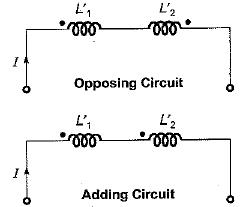Let the self inductances of two coils be  L'and L'2 respectively.
Then, L1 = L'1 + L'2 - 2M (For opposing)
and L2 = L'1 +  L'2  + 2M (For adding)
So, L2 - L1 = 4M
or,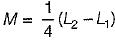QUESTION: 2

Solution:
QUESTION: 3

### In series circuit shown below, for series resonant frequency of 1 rad/s, the value of coupling coefficient K will be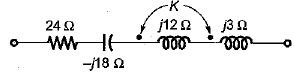Solution:

Using coupling coefficient formula, wo have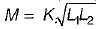Now, for resonance,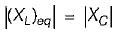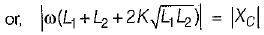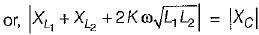or,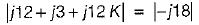or, 15 + 12 k = 18
or, K = 3/12 = 0.25

QUESTION: 4

The equivalent inductance for the following inductive circuit is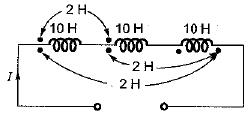Solution:

Leq = (10 + 2 - 2) + (10 + 2 - 2)+ (10 + 2 - 2) = 30 H

QUESTION: 5

The resonant frequency of the given series circuit is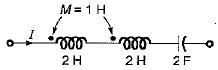Solution:

Leq = L + L + 2 M
= 2 + 2 + 2 x 1 = 6 H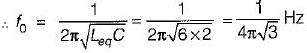QUESTION: 6

A 3 H inductor has 1000 turns. What should be the number of turns to increase the inductance to 5 H?

Solution:

We know that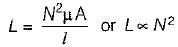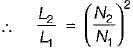or,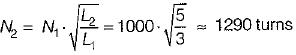QUESTION: 7

What is the equivalent inductance between the terminals A and B for the circuit shown below? (Given, M = 1H)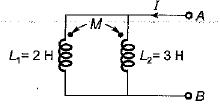Solution:

Due to same polarity, the effect of mutual inductance will he positive.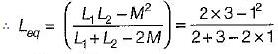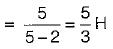QUESTION: 8

Assertion (A): To determine the relative polarity of the induced voltage in the coupled coil, the coils are marked with dots.
Reason (R): One each coil, a dot is placed at the terminals which are instantaneously of the same polarity on the basis of mutual inductance alone.

Solution:
QUESTION: 9

Assertion (A): When a current changes in a circuit, the magnetic fiux linking the same circuit changes and an emf is induced in the circuit.
Reason (R): Mutual inductances is the bilateral property of the linked circuit.

Solution:

The reason for assertion is that, emf is induced due to Faraday’s law of electromagnetic induction. Reason (R) and assertion (A) are individually true.

QUESTION: 10

Match List- I (Connection) with List-ll (Equivalent inductance) and select the correct answer using the codes given below the lists: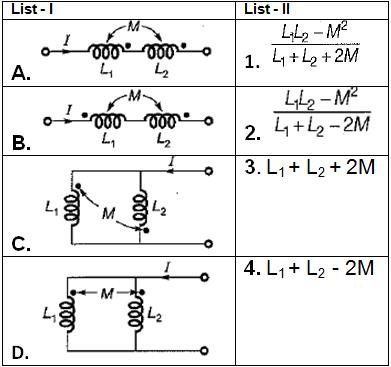Codes: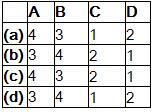Solution: